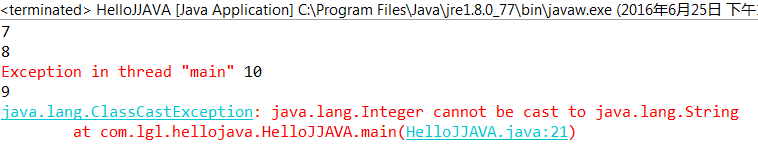# JAVA之旅（二十一）——泛型的概述以及使用，泛型类，泛型方法，静态泛型方法，泛型接口，泛型限定，通配符JAVA之旅（二十一）——泛型的概述以及使用，泛型类，泛型方法，静态泛型方法，泛型接口，泛型限定，通配符http://www.bieryun.com/2796.html## 一.泛型的概述

``````package com.lgl.hellojava;

import java.util.ArrayList;
import java.util.Iterator;

//公共的   类   类名
public class HelloJJAVA {
public static void main(String[] args) {
/**
* 泛型
*/
ArrayList al = new ArrayList();
//添加int
Iterator iterator = al.iterator();
while (iterator.hasNext()) {
String s = (String) iterator.next();
// 打印长度
sop(s.length());
}
}

// 输出
public static void sop(Object obj) {
System.out.println(obj);

}
}
``````• 泛型：JDK1.5版本之后出现的新特性，用于解决安全问题，是一个安全机制；

``ArrayList<String> al = new ArrayList<String>();``

• 好处：讲运行时期出现的问题转移到了编译时期，方便程序员解决问题，让运行时期问题减少，安全

## 二.泛型的使用

``````package com.lgl.hellojava;

import java.util.Comparator;
import java.util.Iterator;
import java.util.TreeSet;

//公共的   类   类名
public class HelloJJAVA {
public static void main(String[] args) {
TreeSet<String> tr = new TreeSet<String>(new LenComp());

Iterator<String> iterator = tr.iterator();
while (iterator.hasNext()) {
String string = iterator.next();
sop(string);
}
}

// 输出
public static void sop(Object obj) {
System.out.println(obj);

}
}

// 比较器
class LenComp implements Comparator<String> {

@Override
public int compare(String o1, String o2) {
String s1 = o1;
int num = new Integer(o1.length()).compareTo(new Integer(o2.length()));
if (num == 0) {
return o1.compareTo(o2);
}
return num;
}

}``````

## 三.泛型类

``````package com.lgl.hellojava;

//公共的   类   类名
public class HelloJJAVA {
public static void main(String[] args) {
Tool t = new Tool();
t.setW(new Worker());
t.getW();
}

// 输出
public static void sop(Object obj) {
System.out.println(obj);

}
}
//操作工人的工具类
class Tool {
private Worker w;

public Worker getW() {
return w;
}

public void setW(Worker w) {
this.w = w;
}

}

class Worker {

}

``````

``````//操作工人的工具类
class Tool {
private Object obj;

public Object getObj() {
return obj;
}

public void setObj(Object obj) {
this.obj = obj;
}

}``````

``````class Utils<T> {

private T t;

public T getT() {
return t;
}

public void setT(T t) {
this.t = t;
}
}``````

• 什么时候定义泛型类

当类中要操作的引用数据类型不确定的时候，早起定义Object来完成扩展，现在定义泛型来完成扩展

## 四.泛型方法

``````package com.lgl.hellojava;

//公共的   类   类名
public class HelloJJAVA {
public static void main(String[] args) {
Demo<String> d = new Demo<String>();
d.show("hh");
d.print("aa");
}
}

class Demo<T> {
public void show(T t) {
System.out.println("show:" + t);
}

public void print(T t) {
System.out.println("print:" + t);
}
}``````

• 为了让不同的方法可以操作不同的类型，而且类型不确定，那么可以将泛型定义在方法上

``````class Demo {
public <T> void show(T t) {

}
public <T>void print(T t){

}
}``````

## 五.静态泛型方法

``````
class Demo<T> {
public <T> void show(T t) {

}

public <T> void print(T t) {

}

public static <T> void method(T t) {

}
}``````

## 六.泛型接口

``````package com.lgl.hellojava;

//公共的   类   类名
public class HelloJJAVA {
public static void main(String[] args) {
InterImpl<Integer> i = new InterImpl<Integer>();
i.show(55);
}
}

class InterImpl<T> implements Inter<T> {

@Override
public void show(T t) {
System.out.println("show:" + t);
}

}

interface Inter<T> {
void show(T t);
}
``````

## 七.泛型限定

``````package com.lgl.hellojava;

import java.util.ArrayList;
import java.util.Iterator;

//公共的   类   类名
public class HelloJJAVA {
public static void main(String[] args) {
ArrayList<String> al = new ArrayList<String>();

ArrayList<Integer> all = new ArrayList<Integer>();

printColl(all);
}
//内容不明确
public static void printColl(ArrayList<?> als) {
Iterator<?> iterator = als.iterator();
while (iterator.hasNext()) {
System.out.println(iterator.next());
}
}
}
``````

``````package com.lgl.hellojava;

import java.util.ArrayList;
import java.util.Iterator;

//公共的   类   类名
public class HelloJJAVA {

public static void main(String[] args) {
ArrayList<Person> al = new ArrayList<Person>();

ArrayList<Student> al1 = new ArrayList<Student>();

// ArrayList
printColl(al1);
}

// 内容不明确
public static void printColl(ArrayList<? extends Person> als) {
Iterator<? extends Person> iterator = als.iterator();
while (iterator.hasNext()) {
System.out.println(iterator.next().getName());
}
}
}

class Person {
private String name;

public Person(String name) {
this.name = name;
}

public String getName() {
return name;
}

public void setName(String name) {
this.name = name;
}
}

class Student extends Person {

public Student(String name) {
super(name);
}
}``````

• ？叫通配符，也可以理解为占位符
• 泛型的限定 ？ extends E:E类型和E的子类型。这叫上限
• ？ super E:可以接受E类型和E的父类，这叫下限• 回顶